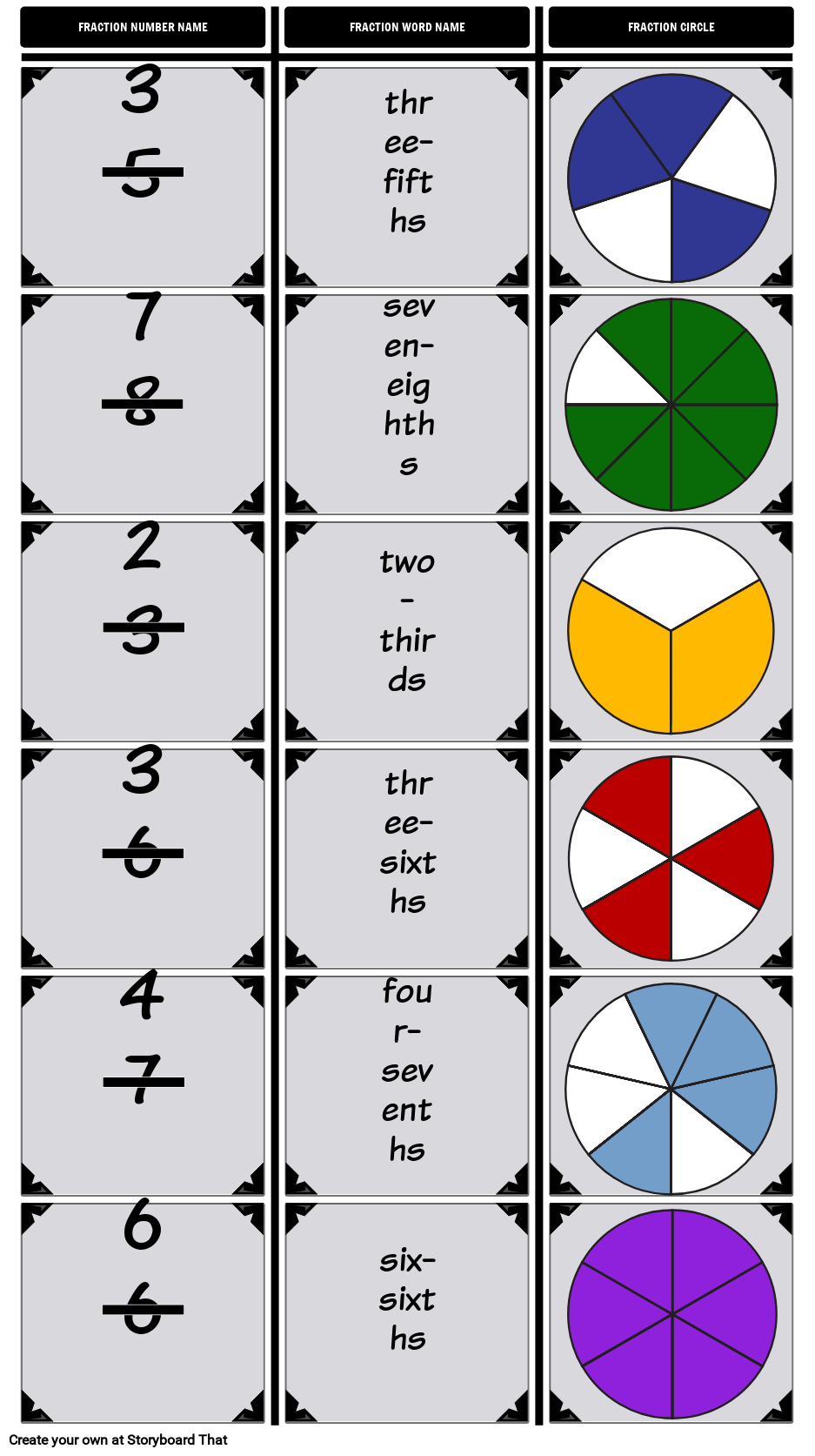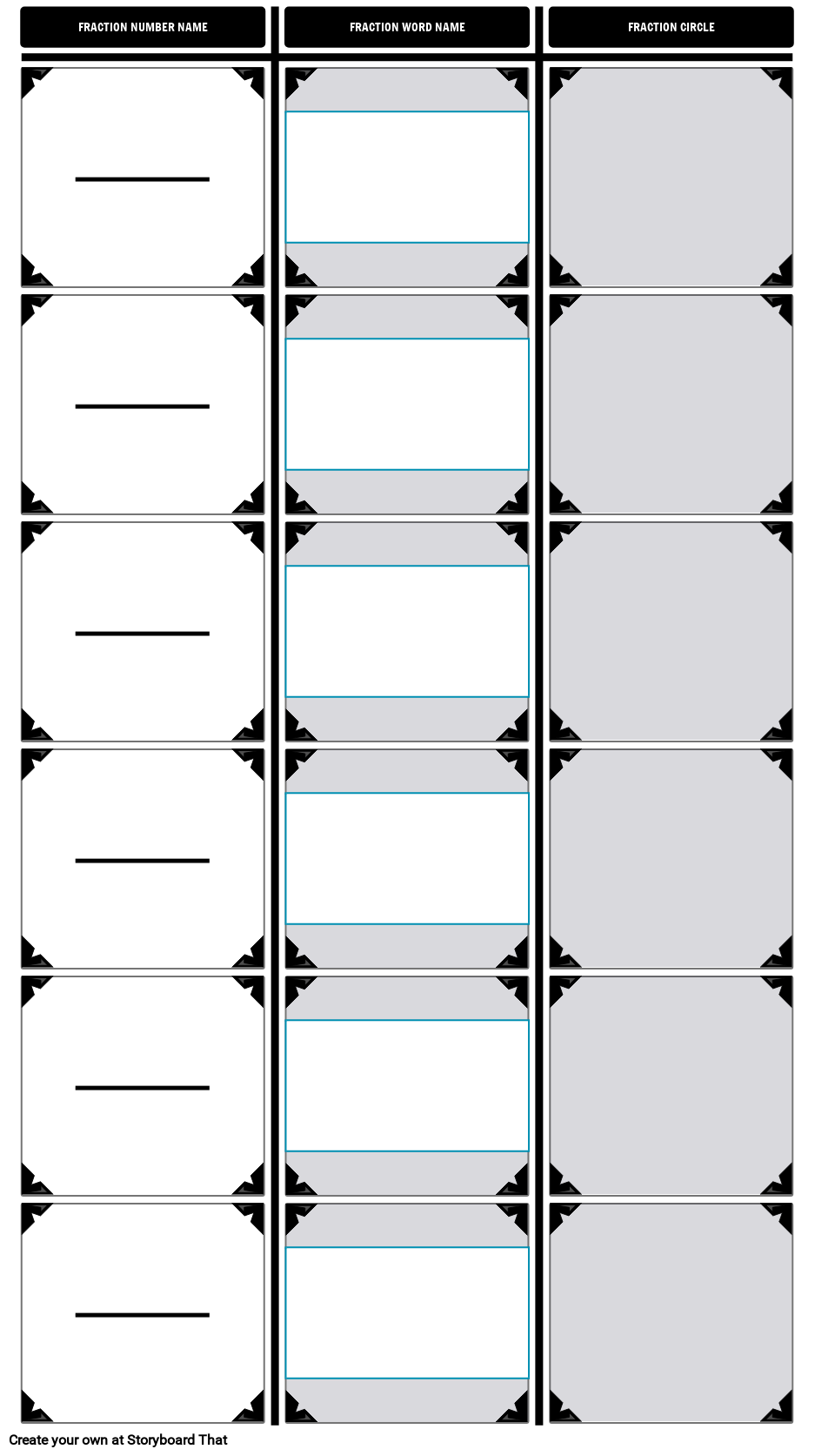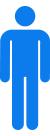ID Fraction Names & Fraction Word Name## Activity Overview

Before doing this activity, introduce the vocabulary of numerator and denominator. The numerator is the number on top of the fraction bar that represents a part of a whole. The denominator is the number below the fraction bar that shows the number of pieces or partitions in a whole. Numerator looks a little like “number” (how many) and denominator may remind some students of “name”, particularly if they are familiar with other languages, such as French or Spanish. The denominator gives the fraction its name (eg. fifths), and the numerator tells you how many parts of the whole there are (three-fifths).

In this activity, students will identify the given fractions and fraction pictures by both number and word names. Students can also use spider maps for this assignment, although the template provided uses a T-Chart. Depending on the level of complexity, have students fill in different columns, such as providing them with a few shapes and a few fraction word names and having students fill in the blanks.

Lesson Plan Reference

Difficulty Level 3 (Developing to Mastery)

Type of Assignment Individual or Partner

Common Core Standards
• [Math/Content/3/NF/A/1] Understand a fraction 1/b as the quantity formed by 1 part when a whole is partitioned into b equal parts; understand a fraction a/b as the quantity formed by a parts of size 1/b.

## Template and Class Instructions

(These instructions are completely customizable. After clicking "Use This Assignment With My Students", update the instructions on the Edit Tab of the assignment.)

#### Student Instructions

Practice the multiple ways you can show fractions: the fraction number name, the fraction word name, and as a fraction circle.

1. Choose six different fractions. (Denominators can only be 1-8.)
2. Type in the fraction number names in the first column.
• Click on the fraction bar to activate the text.
3. Type in the fraction word name in the second column.
4. Add the correct fraction circle to the third column.
• Fraction circles are located in the shapes category.
5. Change the colors of the fraction circles to match the number and word names.
ID Fraction Name Template## Education Pricing

This pricing structure is only available to academic institutions. Storyboard That accepts purchase orders.#### Single Teacher

As low as /month#### Department

As low as /month#### School/District

As low as /month

*(This will start a 2-Week Free Trial - No Credit Card Needed)
##### Over 15 Million Storyboards Created